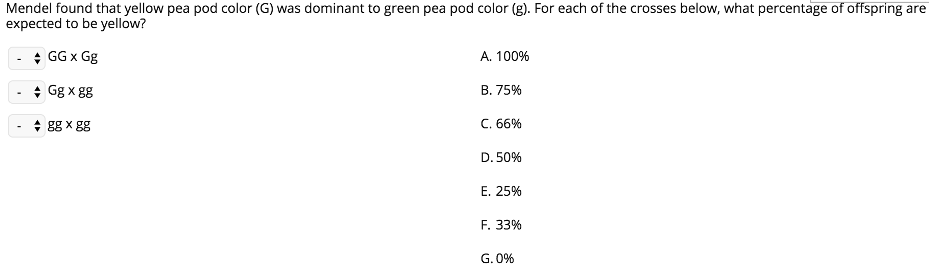# Problem: Mendel found that yellow pea pod color (G) was dominant to green pea pod color (g). For each of the crosses below, what percentage of offspring are expected to be yellow?I. GG x Gg          II. Gg x gg          III. gg x ggA. 100%B. 75%C. 66%D. 50%E. 25%F. 33%G. 0%

###### FREE Expert Solution

In the given problem, it can be seen that each individual carries two alleles for the gene. It is thus safe to assume that the individuals are diploids. For a cross between these kinds of organisms, it would be expected that there are four outcomes if only a single gene is being considered.###### Problem Details

Mendel found that yellow pea pod color (G) was dominant to green pea pod color (g). For each of the crosses below, what percentage of offspring are expected to be yellow?

I. GG x Gg          II. Gg x gg          III. gg x gg

A. 100%

B. 75%

C. 66%

D. 50%

E. 25%

F. 33%

G. 0%